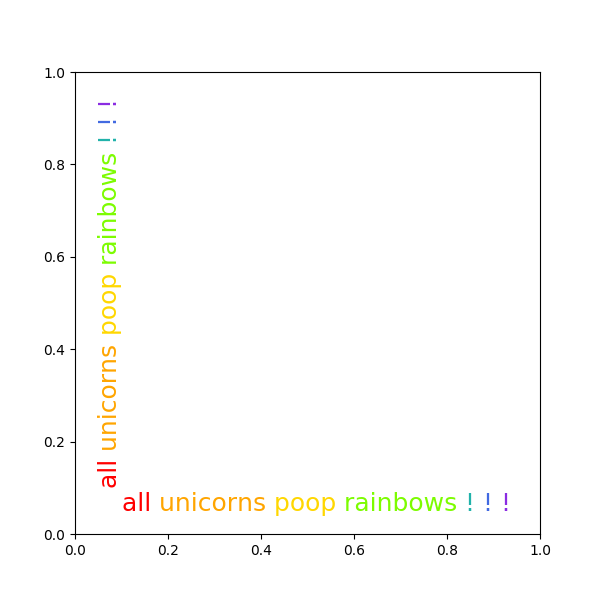# Rainbow text¶

The example shows how to string together several text objects.

## History¶

On the matplotlib-users list back in February 2012, Gökhan Sever asked the following question:

Is there a way in matplotlib to partially specify the color of a string?

Example:

plt.ylabel("Today is cloudy.")

How can I show "today" as red, "is" as green and "cloudy." as blue?

Thanks.

Paul Ivanov responded with this answer:import matplotlib.pyplot as plt
from matplotlib import transforms

def rainbow_text(x, y, strings, colors, orientation='horizontal',
ax=None, **kwargs):
"""
Take a list of *strings* and *colors* and place them next to each
other, with text strings[i] being shown in colors[i].

Parameters
----------
x, y : float
Text position in data coordinates.
strings : list of str
The strings to draw.
colors : list of color
The colors to use.
orientation : {'horizontal', 'vertical'}
ax : Axes, optional
The Axes to draw into. If None, the current axes will be used.
**kwargs
All other keyword arguments are passed to plt.text(), so you can
set the font size, family, etc.
"""
if ax is None:
ax = plt.gca()
t = ax.transData
canvas = ax.figure.canvas

assert orientation in ['horizontal', 'vertical']
if orientation == 'vertical':
kwargs.update(rotation=90, verticalalignment='bottom')

for s, c in zip(strings, colors):
text = ax.text(x, y, s + " ", color=c, transform=t, **kwargs)

# Need to draw to update the text position.
text.draw(canvas.get_renderer())
ex = text.get_window_extent()
if orientation == 'horizontal':
t = transforms.offset_copy(
text.get_transform(), x=ex.width, units='dots')
else:
t = transforms.offset_copy(
text.get_transform(), y=ex.height, units='dots')

words = "all unicorns poop rainbows ! ! !".split()
colors = ['red', 'orange', 'gold', 'lawngreen', 'lightseagreen', 'royalblue',
'blueviolet']
plt.figure(figsize=(6, 6))
rainbow_text(0.1, 0.05, words, colors, size=18)
rainbow_text(0.05, 0.1, words, colors, orientation='vertical', size=18)

plt.show()


Keywords: matplotlib code example, codex, python plot, pyplot Gallery generated by Sphinx-Gallery